The First Personality Reading Based On
The Sacred Geometry Of Your Name Symbols.

# Numerology numbers

Hidden meaning of numbers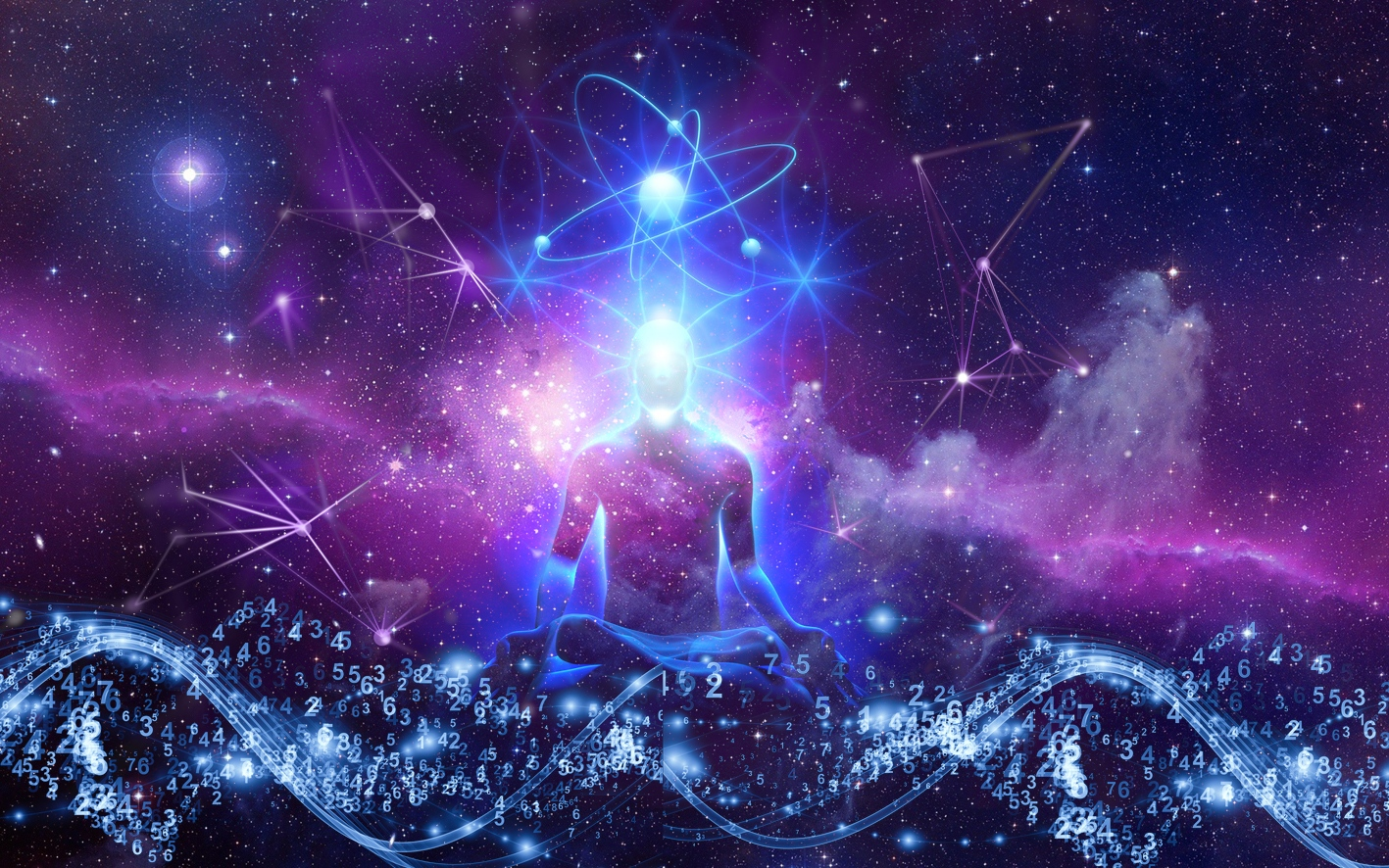## First elements of numbers personality

Number Features

The Pythagoreans are the first to point out the Pairs of Opposites that function in nature and in man; they teach that all things are composed of opposites or opposites: one and multiple, limited and unlimited, odd and even, male and female, rest and movement, light and darkness.

From its mathematical qualities, the Pythagoreans established a link between Numbers and Ideas.

«  This science of numbers was known by various names in the temples of Egypt and Asia. Since it provided the key to all doctrine, it was carefully hidden from the common people. The numbers, letters, geometric figures, or human representations that served as signs for this algebra of the occult world were understood only by the initiated. The latter discovered the meaning to the followers only after having received the oath of silence. Pythagoras formulated this science in a book written in his own hand called Hieros Logos, the sacred word. »

Edouard Schuré ~ Les Grands-Initiés

### Even and odd numbers

Odd numbers are considered masculine and active while even numbers are considered feminine and receptive.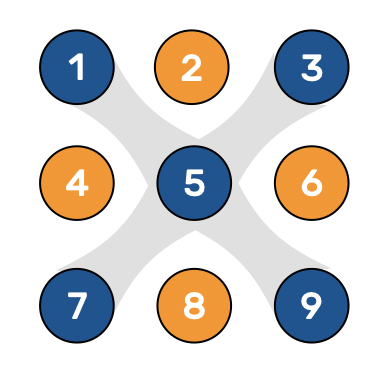We will see later that this gender classification is no longer valid according to Source Numbers of Geonumerology.

For example the number 4 obtained by 13 = 1 + 3 is a tetrahedron, symbol of fire and thus masculine; while the number 4 obtained by 22 = 2 + 2 is visualized in the form of a cross, of a female nature.

### Complementary numbers

Numbers located at the extremities and whose sum makes 10: 1+9, 2+8, 3+7, 4+6, 5+5, as well as their symmetrical: 64, 73, ...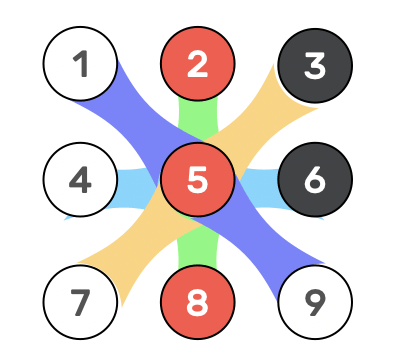This aspect is essentially interesting when studying a geonumerologic profile. Having each 5 personal symbols plus the life path symbol, it is possible to have several complementary numbers in profile.

This is extremely beneficial since they have the advantage of recreating a unit.

### Representative numbers

Numbers keeping their place whatever the layout: in line or in column. The number has of the same color as its World.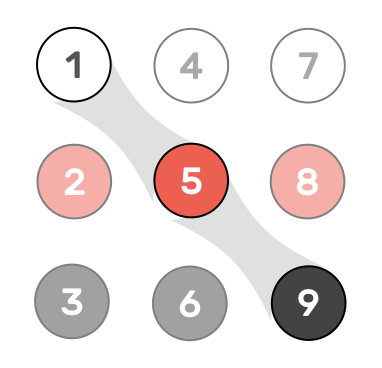These three numbers (159) are very important because they give us the key of the Three World: Dive, Human and Natural or Spiritual, Psychological and Natural.

The 6 others are considered as "reflections" (from one world to another).

These are the occult relations linking numbers with the same secret value calculated by reducing triangular numbers.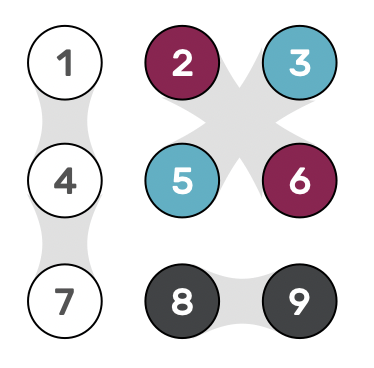The secret value of numbers brings to light hidden relationships between the first 9 numbers and in particular the seriality of the unit evolving through the three planes.

## Reduction & decomposition

The 29 unique ways to obtain the nine reduced numbers

#### Reduction to 9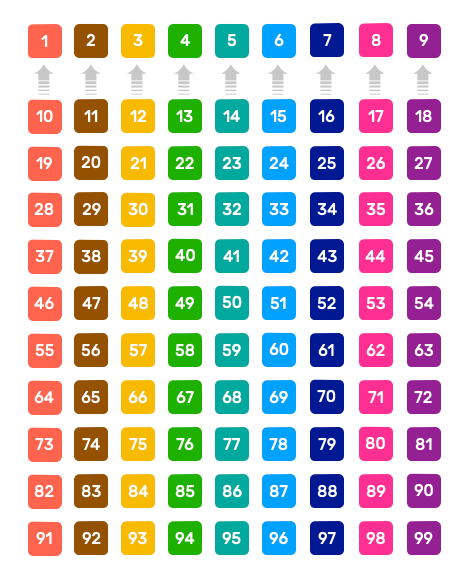All the numbers in a column have the same reduction (indicated in the first row with the first 9 sigle-digit numbers).
Ex : number 58. Its reduction is 5 + 8  = 13 and 13 = 1 + 3 = 4.

#### Decomposition to 29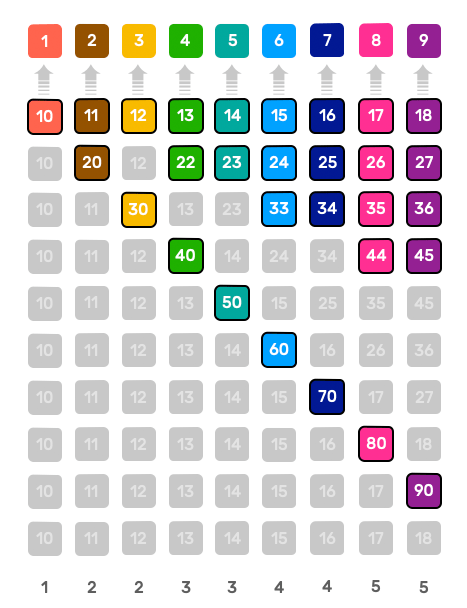Numbers in gray represent duplicates.
We see that apart from the number one all other numbers can be obtained in different ways. The reduced number 4 has three source numbers : 13, 22 & 40.

By studying how all numbers are reduced to the first nine, geonumerology identifies 29 unique possibilities. While all the numbers in a column have the same reduction-number (first row of the table), not all do so in the same way.

We can see that the reduction of a number can be obtained in several ways (except for 1).

If we take the example of the number 7 in the table on the left, we can see that there are four unique possibilities (without the duplicates: greyed cells) to obtain the number 7:

• 1 + 6 (or symmetrical sound 61)
• 2 + 5 (or symmetrical sound 52)
• 3 + 4 (or symmetrical sound 43)
• 7 + 0

The approach followed by Geonumerology is similar to the process of Alchemy. After having condensed the infinity of numbers into a 9-box matrix, we will now study the ways to obtain these first 9 numbers.

To do this, we will identify the decomposition that precedes the reduced number.

Knowing a number means first of all relating it to other numbers from which it necessarily proceeds, to other elements that determine its formation beforehand.

« Thus the idea of any integer is essentially composed by the multiplication of the simple idea, and the degree of its composition or product is measured by the number of units it contains, or by the rank it occupies in the infinite scale of numbers.  »

De l'Unité ~ Martin Etchegoyen

## The 29 Source-Numbers

The 29 personality archetypes.

The 29 Source Numbers of geonumerology each correspond to an equation, a formula, which gives us the keys to its geometrical construction : crosses, polygons, solids, figurate numbers, etc.

There are three major types of source numbers:

• Solitary numbers of type X = XO (examples; 1=10, 2=20, 3=30, ...). These numbers correspond to the polygons of the circle;
• Dual numbers of type X = YY (examples; 2=11, 4=22, 6=33 and 8=44). These numbers correspond to the dual numbers;
• Compound numbers of type X = YZ (examples; 3=12, 4=13, 5=14, etc). Concerns the 15 other numbers named compound numbers.

To know a number, it is first of all to link it to other previous numbers from which it necessarily proceeds, to the conditions which determine its formation beforehand.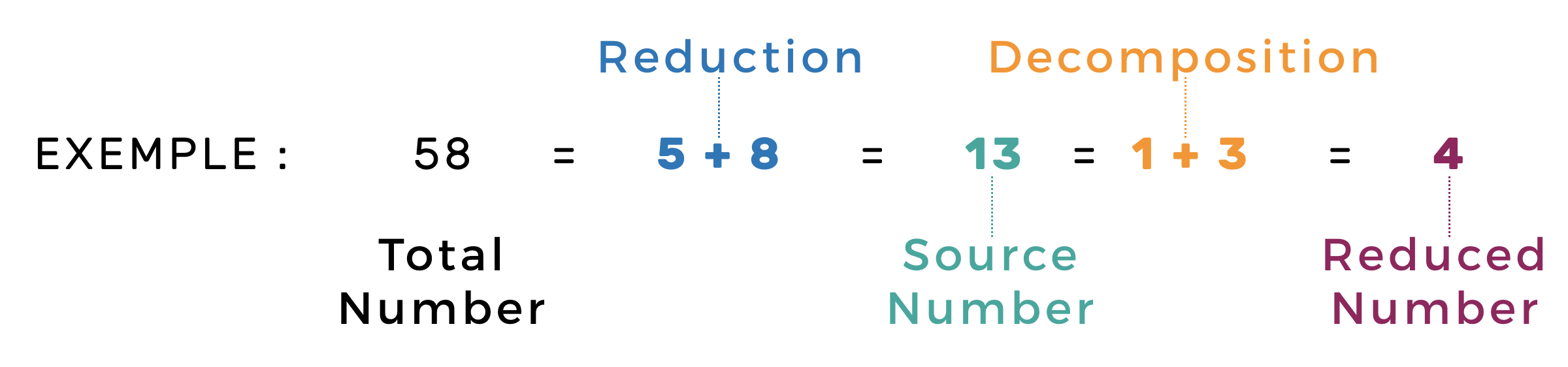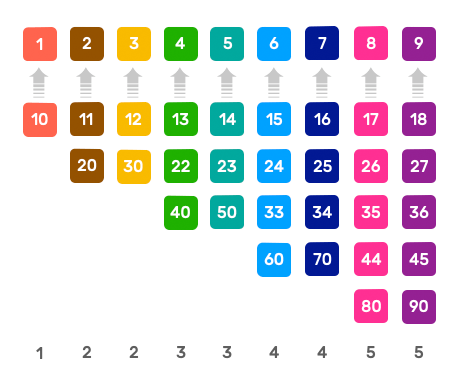In the example on the left, the Number 4 obtained from the total number 58 derives from the source number 13 whose decomposition is 1 + 3.

#### ↙︎Morphology

Shows us HOW the number is made up,

as well as the nature of its components

#### ⇩Psychology

Personality's Traits and Types

#### ↘︎Topology

Tells us WHERE the number is located in the matrix,

in which World: Spiritual, Psychic or Physical.

Meaning of life

## Matrix Typology

The Spirit of the place

In the matrices below, each source number is represented with its components. Thus for example the number 2 obtained by 11 or 1 + 1 = 2 will occupy two cells in the matrix: those of numbers 1 and 2.

You can see that there are multiple differences between the 29 source numbers. Solitary numbers, for example, occupy only one cell (e.g. 10 = 1 + 0 - only the cell of number 1 is "active"). On the other hand, the compound number 13 which gives 4 by 1 + 3 occupies three cells.

Some numbers have the particularity of being present in all the three Worlds, which makes them particularly "integrated" numbers. This is the case for example of the numbers 16, 25, 34, ...

This typology will be useful to us during the spiritual interpretation of a profile in particular according to the location of the number of expression as well as the path of life number.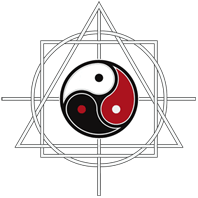THE SACRED GEOMETRY OF YOUR NAME